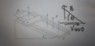# Find induced current, magnetic force, work in inclined plane

• Helly123

## Homework Statement

http://[url=https://ibb.co/b3Emfo]https://preview.ibb.co/gLaEY8/20180527_055248.jpg[ [ATTACH=full]226218[/ATTACH] A conducting bar slides down without friction on a pair of conducting rails separated by distance d. Connected to resistor R and there magnetic field B directed upward, perpendicular to the ground over the entire region through which the bar moves.
1) find the current
2) find the formula for magnetic force
3) After sufficient of time bar moves at constant speed. At this terminal velocity u the gravity force is balanced by the magnetic force along the inclined plane
Find correct formula for u?
4) what is the formula for rate of work done by gravity on the bar?

[h2]Homework Equations[/h2]
I = emf/R
I = (vBLsin ##\theta## )/R

[h2]The Attempt at a Solution[/h2]
1) current
I = emf/R
I = (vBLsin ##\theta## )/R
I = ( v cos ##\theta## B L sin 90)/R

Is B perpendicular to ground? Not to the inclined plane?
So we need v cos theta which perpendicular to B, and makes sin theta to sin 90 ?

2) Find formula for magnetic force
F = IBL sin 90
Why ##cos^2\theta##? Because
The current in 1) after calculation with v cos ##\theta## is perpendicular to B that is I cos ##\theta## ?

3)
Could it be gravity forced balanced by F sin ##\theta## ?
Do we have to find u = velocity?
If yes. How is velocity and force related?

4)
W = mg
##\Sigma##F = m.a

#### Attachments

•20180527_055248.jpg
11.4 KB · Views: 813
Last edited:

•